# ACIDS AND BASES Calculating the pH at equivalence of a titration A chemist titrates 200.0 mL...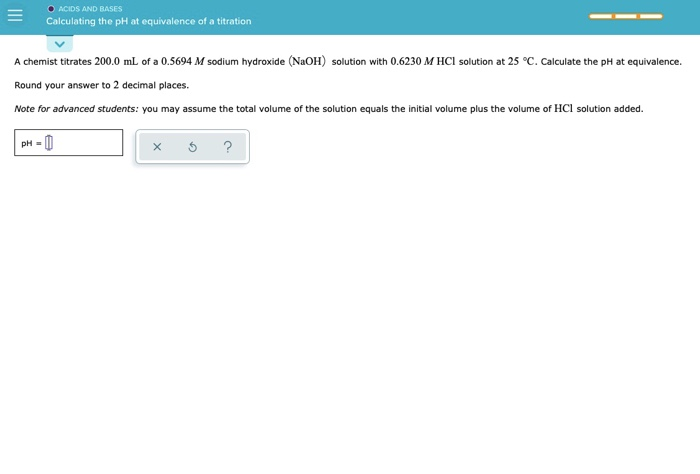ACIDS AND BASES Calculating the pH at equivalence of a titration A chemist titrates 200.0 mL of a 0.5694 M sodium hydroxide (NaOH) solution with 0.6230 M HCl solution at 25 °C. Calculate the pH at equivalence. Round your answer to 2 decimal places. Note for advanced students: you may assume the total volume of the solution equals the initial volume plus the volume of HCl solution added. PH-0 5 ?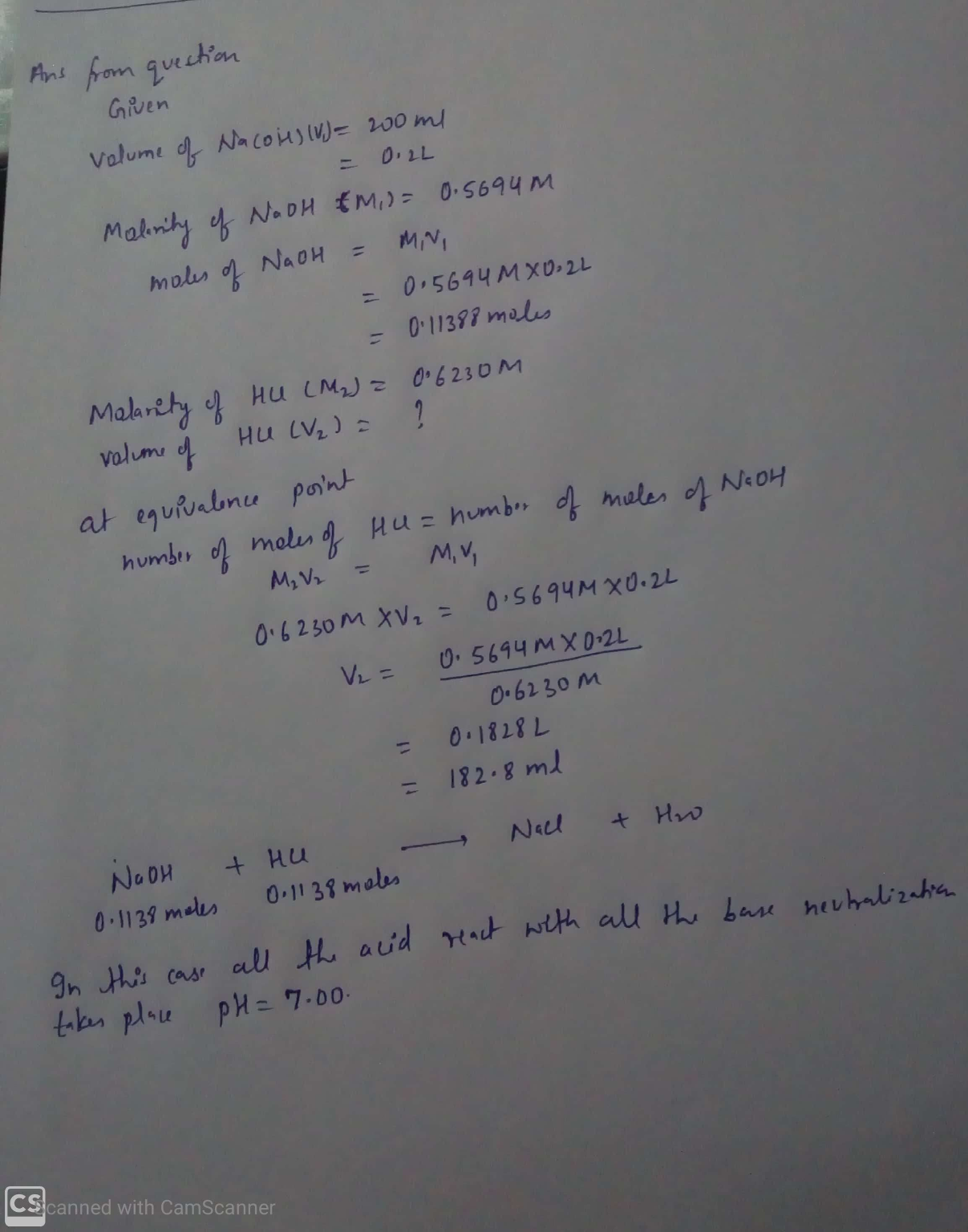#### Earn Coin

Coins can be redeemed for fabulous gifts.

Similar Homework Help Questions
• ### = O ACIDS AND BASES Calculating the pH at equivalence of a titration A chemist titrates...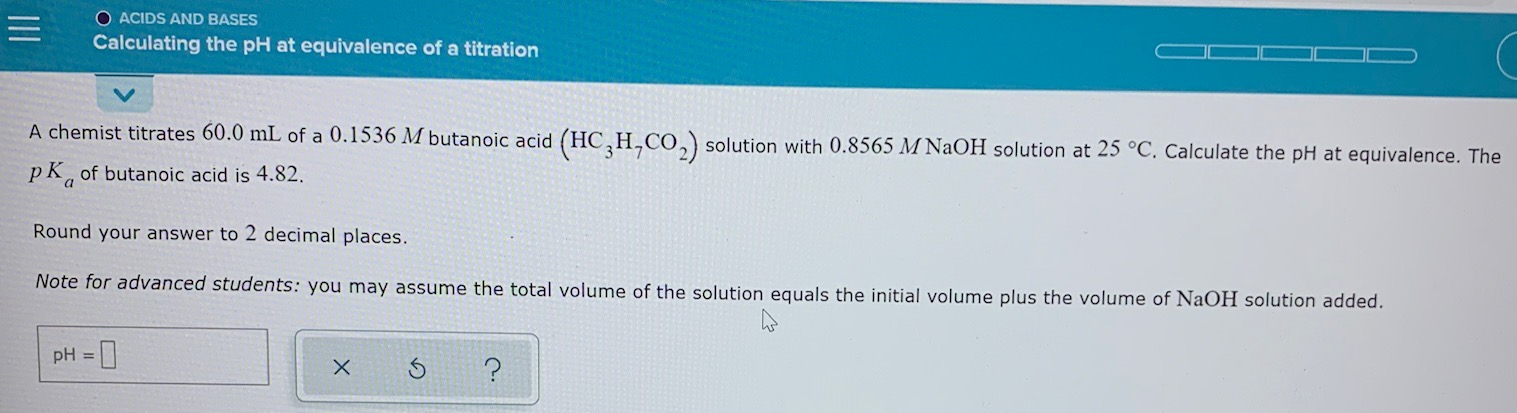= O ACIDS AND BASES Calculating the pH at equivalence of a titration A chemist titrates 60.0 mL of a 0.1536 M butanoic acid (HC,H,CO2) solution with 0.8565 M NaOH solution at 25 °C. Calculate the pH at equivalence. The pk, of butanoic acid is 4.82. Round your answer to 2 decimal places. Note for advanced students: you may assume the total volume of the solution equals the initial volume plus the volume of NaOH solution added. pH = x...

• ### ACIDS AND BASES Calculating the pH at equivalence of a titration A chemist titrates 130.0 mL...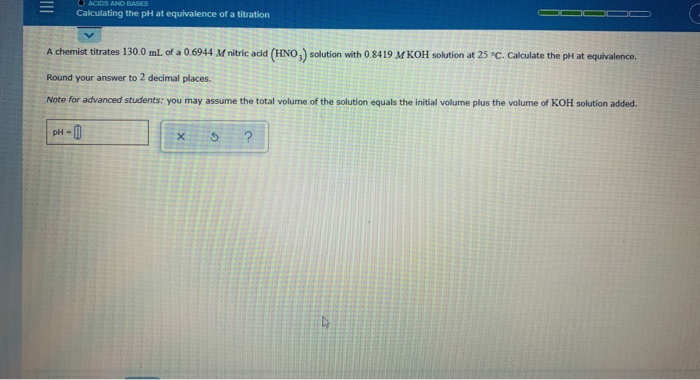ACIDS AND BASES Calculating the pH at equivalence of a titration A chemist titrates 130.0 mL of a 0.6944 M nitric acid (HNO.) solution with 0.8419 M KOH solution at 25 °C. Calculate the pH at equivalence Round your answer to 2 decimal places. Note for advanced students: you may assume the total volume of the solution equals the initial volume plus the volume of KOH solution added. pH-0 X 5 ?

• ### - Calculating the pH at equivalence of a titration A chemist titrates 180.0 ml of a...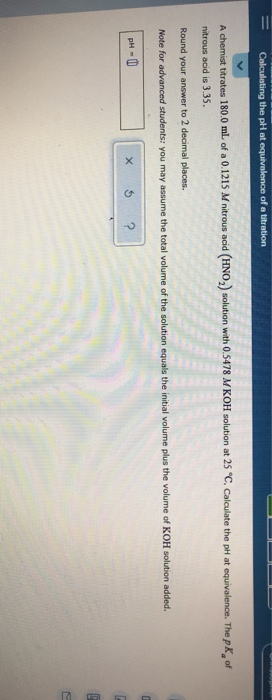- Calculating the pH at equivalence of a titration A chemist titrates 180.0 ml of a 0.1215 M nitrous acid (HNO) solution with 0.5478 M KOH solution at 25 "C. Calculate the pH at equivalence. The pk of nitrous acid is 3.35. Round your answer to 2 decimal places. Note for advanced students: you may assume the total volume of the solution equals the initial volume plus the volume of KOH solution added. PHU X 5 ?

• ### Calculating the pH at equivalence of a titration A chemist titrates 120.0 mL of a 0.7513...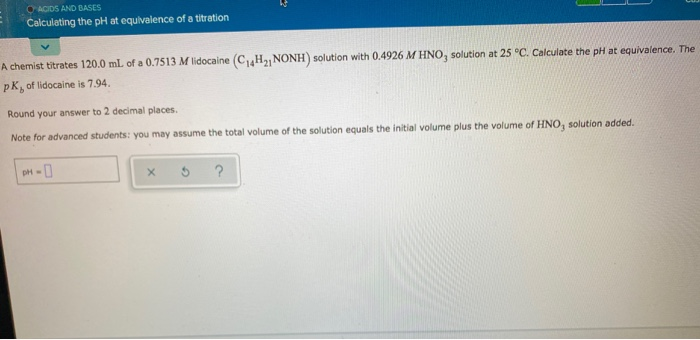Calculating the pH at equivalence of a titration A chemist titrates 120.0 mL of a 0.7513 M lidocaine (C4H2, NONH) solution with 0.4926 M HNO, solution at 25°C. Calculate the pH at equivalence. The PK, of lidocaine is 7.94 Round your answer to 2 decimal places Note for advanced students: you may assume the total volume of the solution equals the initial volume plus the volume of HNO, solution added. DH-

• ### A chemist titrates 200.0 mL of a 0.7681 M hydrocyanic acid (HCN) solution with 0.5271 M...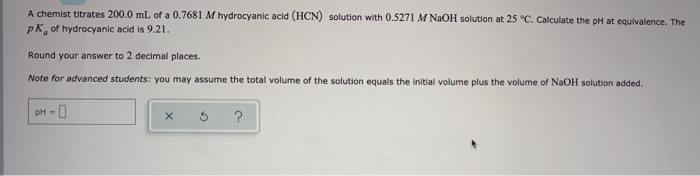A chemist titrates 200.0 mL of a 0.7681 M hydrocyanic acid (HCN) solution with 0.5271 M NaOH solution at 25 °C. Calculate the pH at equivalence. The pk of hydrocyanic acid is 9.21. Round your answer to 2 decimal places. Note for advanced students: you may assume the total volume of the solution equals the initial volume plus the volume of NaOH solution added. pH- х ?

• ### Calculating the pH at equi.. A chemist titrates 70.0 ml. of a 04557 M carbonic acid...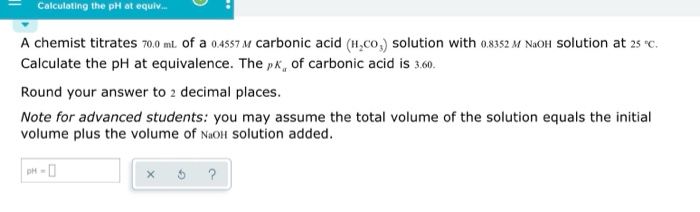Calculating the pH at equi.. A chemist titrates 70.0 ml. of a 04557 M carbonic acid (H,co,) solution with o8352 M NaOlH Solution at 2s c Calculate the pH at equivalence. The pk, of carbonic acid is 3.60 Round your answer to 2 decimal places. Note for advanced students: you may assume the total volume of the solution equals the initial volume plus the volume of NaoH Solution added. рH - D

• ### A chemist titrates 150.0 mL of a 0.1029 M potassium hydroxide (KOH) solution with 0.8645 M...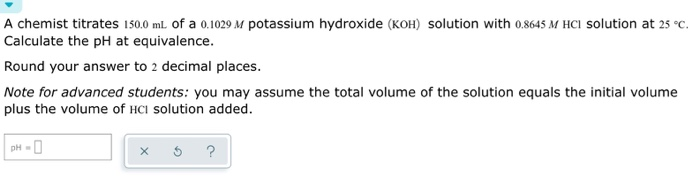A chemist titrates 150.0 mL of a 0.1029 M potassium hydroxide (KOH) solution with 0.8645 M HCl solution at 25°C. Calculate the pH at equivalence. Round your answer to 2 decimal places. Note for advanced students: you may assume the total volume of the solution equals the initial volume plus the volume of HCl solution added. x 5 ?

• ### O ACIDS AND BASES Calculating the pH of a weak ba.. An analytical chemist is titrating...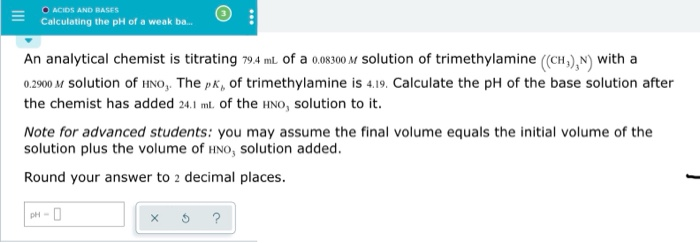O ACIDS AND BASES Calculating the pH of a weak ba.. An analytical chemist is titrating 79.4 ml of a 0.08300 M solution of trimethylamine (CH), N) with a 0.2900 M solution of HNO, The px, of trimethylamine is 4.19. Calculate the pH of the base solution after the chemist has added 24.1 mt of the HNO, solution to it. Note for advanced students: you may assume the final volume equals the initial volume of the solution plus the volume...

• ### = surviving chem 2!! Ah really O ACIDS AND BASES Calculating the pH of a weak...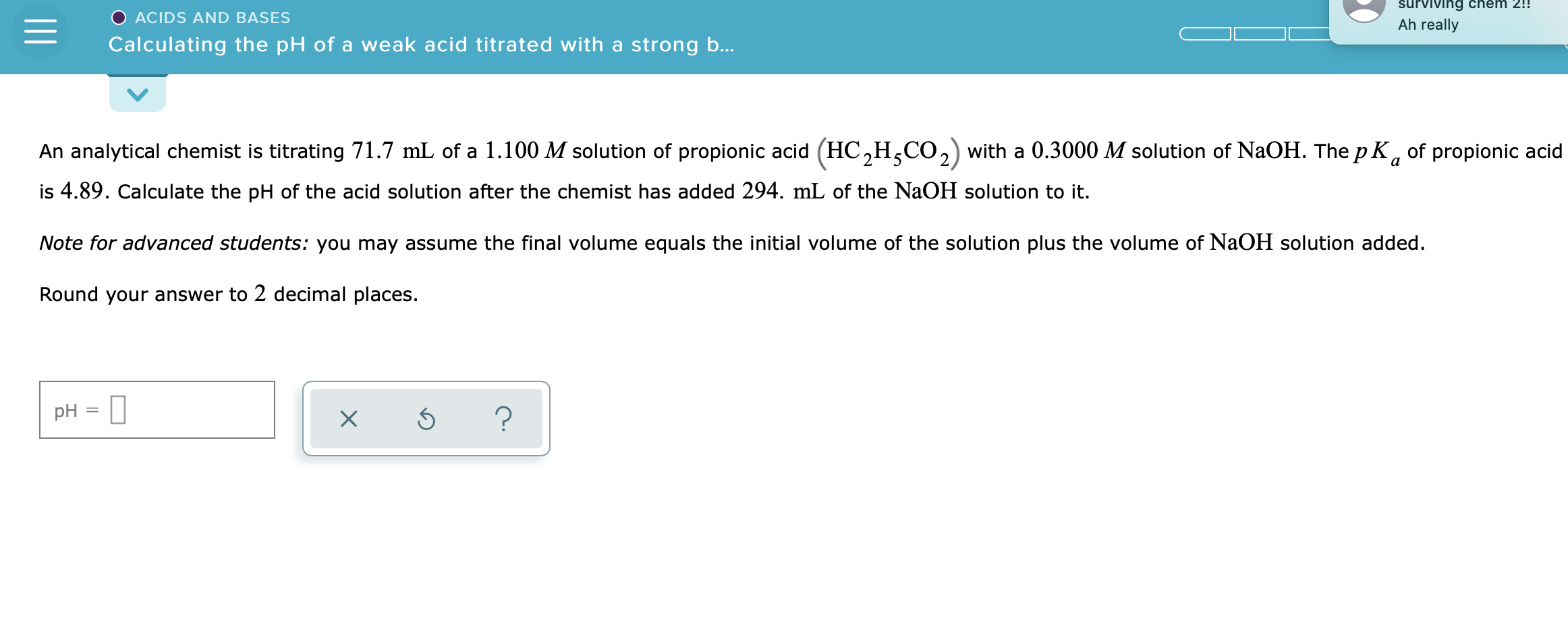= surviving chem 2!! Ah really O ACIDS AND BASES Calculating the pH of a weak acid titrated with a strong b... An analytical chemist is titrating 71.7 mL of a 1.100 M solution of propionic acid (HC,H,CO2) with a 0.3000 M solution of NaOH. The pK, of propionic acid is 4.89. Calculate the pH of the acid solution after the chemist has added 294. mL of the NaOH solution to it. Note for advanced students: you may assume the...

• ### A chemist titrates 80.0 mL of a 0.3371 M ammonia (NH3) solution with 0.5400 M HCl...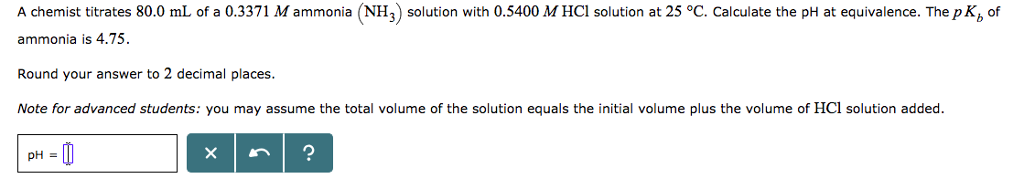A chemist titrates 80.0 mL of a 0.3371 M ammonia (NH3) solution with 0.5400 M HCl solution at 25 °C. Calculate the pH at equivalence. The pK, of ammonia is 4.75. Round your answer to 2 decimal places. Note for advanced students: you may assume the total volume of the solution equals the initial volume plus the volume of HCl solution added.